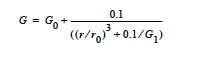# Pseudo-Solid Lame MU#

Pseudo-Solid Lame MU = {model_name} {float_list} [M/Lt2]


## Description / Usage#

This card is required only for TOTAL_ALE mesh motion types (see Mesh Motion card) and is used to specify the model for the Lame coefficient $$\mu$$ for the mesh motion solid constitutive equation (see Sackinger et al. 1995, and Solid Constitutive Equation card); this coefficient is equivalent to the shear modulus G. The model list here is abbreviated as compared to the Lame MU card as these properties are just used to aid in the elastic mesh motion, independent of the material.

Definitions of the input parameters are as follows:

 {model_name} Name of the Lame’ Mu coefficient model. This parameter can have one of the following values: CONSTANT or CONTACT_LINE. {float_list} One or more floating point numbers ( through ) whose values are determined by the selection for {model_name}. These are identified in the discussion of each model below.

The details of each model option are:

 CONSTANT For the CONSTANT model, {float_list} is a single value: - Standard value of the μ (or the shear modulus G for the mesh). See Pseudo Solid Constitutive Equation card. CONTACT_LINE The CONTACT_LINE model is a convenient way to control mesh deformation near a fixed point and is normally used ONLY for TOTAL_ALE or ARBITRARY Mesh Motion types. This model enables the user to make the shear modulus much larger near the contact line (fixed point) than far away from the contact line, so that elements near the contact line are forced to retain their shape. The shear modulus in this model varies inversely with distance from the contact line: This card specifies the mesh motion in the ALE solid region is to conform to the nonlinear elastic model, as described on the Solid Constitutive Equation card. This card is required together with Pseudo-Solid Lame Mu and Pseudo-Solid Lame Lambda cards.r is the distance from the fixed point, $$r_0$$ is a decay length, $$G_0$$ is the modulus far from the contact line, and $$G_0+r_0$$ is the modulus at the contact line. The {float_list} contains four values for this model, where: - Node set number of the fixed point (converted to an integer by Goma) - $$G_0$$ (or $$\mu_0$$) - $$G_1$$ - $$r_0$$

## Examples#

Pseudo-Solid Lame MU = CONSTANT 0.5


This card specifies that the current material have a constant shear modulus of 0.5 for the mesh elasticity. Note that the real-solid mesh Lame MU is set with the Lame MU card.

## Technical Discussion#

It is best to consult the TALE tutorial (Schunk, 1999) for details of this card.